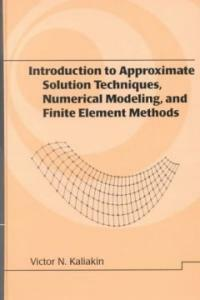> 상세정보

상세정보Introduction to approximate solution techniques, numerical modeling, and finite element methods (2회 대출)

자료유형
단행본
개인저자
Kaliakin, Victor N.
서명 / 저자사항
Introduction to approximate solution techniques, numerical modeling, and finite element methods / Victor N. Kaliakin.
발행사항
New York :   Marcel Dekker,   c2002.
형태사항
xvi, 674 p. : ill. ; 24 cm.
총서사항
Civil and environmental engineering ;9
ISBN
082470679X (hbk. : alk. paper) 9780824706791 (hbk. : alk. paper)
내용주기
1. Governing Equations and Their Approximate Solution -- 2. Computer Storage and Manipulation of Numbers -- 3. The Finite Difference Method -- 4. The Method of Weighted Residuals -- 5. Variational Methods -- 6. Introduction to the Finite Element Method -- 7. Development of Finite Element Equations -- 8. Steps in Performing Finite Element Analyses -- 9. Element Interpolation Functions -- 10. Element Mapping -- 11. Finite Element Analysis of Scalar Field Problems -- 12. Finite Element Analysis in Linear Elastostatics -- 13. Implementation, Modeling, and Related Issues -- A. Mathematical Potpourri -- B. Some Notes on Heat Flow -- C. Local and Natural Coordinate Systems -- D. The Patch Test -- E. Solution of Linear Systems of Equations -- F. Notes on Integration of Finite Elements.
서지주기
Includes bibliographical references (p. 613-663) and index.
일반주제명
Finite element method.
 000 02050camuu2200349 a 4500 001 000045365568 005 20070626105705 008 010724s2002 nyua b 001 0 eng 010 ▼a 2001047122 020 ▼a 082470679X (hbk. : alk. paper) 020 ▼a 9780824706791 (hbk. : alk. paper) 024 3 1 ▼a 9780824706791 035 ▼a (OCoLC)ocm47696342 035 ▼a (OCoLC)47696342 035 ▼a (KERIS)REF000006500253 040 ▼a DLC ▼c DLC ▼d C#P ▼d KKU ▼d OrLoB-B ▼d 211009 042 ▼a pcc 050 0 0 ▼a TA347.F5 ▼b K35 2002 082 0 0 ▼a 620/.001/51535 ▼2 21 082 0 4 ▼a 620.001/51825 ▼2 22 090 ▼a 620.00151825 ▼b K14i 100 1 ▼a Kaliakin, Victor N. 245 1 0 ▼a Introduction to approximate solution techniques, numerical modeling, and finite element methods / ▼c Victor N. Kaliakin. 260 ▼a New York : ▼b Marcel Dekker, ▼c c2002. 300 ▼a xvi, 674 p. : ▼b ill. ; ▼c 24 cm. 440 0 ▼a Civil and environmental engineering ; ▼v 9 504 ▼a Includes bibliographical references (p. 613-663) and index. 505 0 ▼a 1. Governing Equations and Their Approximate Solution -- 2. Computer Storage and Manipulation of Numbers -- 3. The Finite Difference Method -- 4. The Method of Weighted Residuals -- 5. Variational Methods -- 6. Introduction to the Finite Element Method -- 7. Development of Finite Element Equations -- 8. Steps in Performing Finite Element Analyses -- 9. Element Interpolation Functions -- 10. Element Mapping -- 11. Finite Element Analysis of Scalar Field Problems -- 12. Finite Element Analysis in Linear Elastostatics -- 13. Implementation, Modeling, and Related Issues -- A. Mathematical Potpourri -- B. Some Notes on Heat Flow -- C. Local and Natural Coordinate Systems -- D. The Patch Test -- E. Solution of Linear Systems of Equations -- F. Notes on Integration of Finite Elements. 650 0 ▼a Finite element method. 945 ▼a KINS

소장정보

No. 소장처 청구기호 등록번호 도서상태 반납예정일 예약 서비스
No. 1 소장처 청구기호 620.00151825 K14i 등록번호 121148681 도서상태 대출가능 반납예정일 예약 서비스

컨텐츠정보

목차

Governing equations and their approximate solution; computer storage and manipulation of numbers; the finite difference method; the method of weighted residuals; variational methods; introduction to the finite element method; development of finite element equations; steps in performing finite element analysis; element interpolation functions; element mapping; finite element analysis of scalar field problems; finite element analysis in linear elastostatics; implementation, modelling, and related issues. Appendices: mathematical potpourri; matrices and linear algebra; some notes on heat flow; local and natural coordinate systems; the patch test; solution of linear systems of equations; notes on integration of finite elements.

관련분야 신착자료

솔리드이엔지 (2021)

(Python을 이용한) 공학수치해석 / 수정판

Kiusalaas, Jaan (2021)

이상만 (2021)

김정원 (2021)

마스터링 리버스 엔지니어링 : 소프트웨어 취약점을 분석하는 해커 친화적 기술

Wong, Reginald (2021)

Nanopore technology : methods and protocols

Fahie, Monifa A. V (2021)

김흥수 (2021)

기계재료 편람 : 금속 및 비금속재료 KS규격 핸드북

테크노공학기술연구소 (2021)